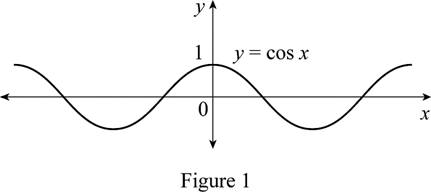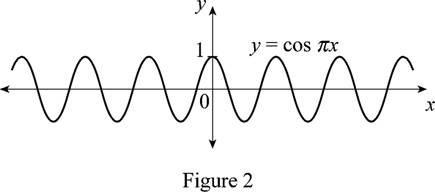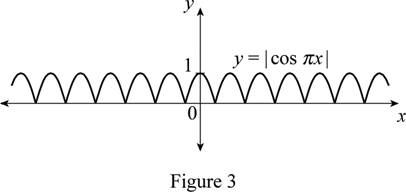# The function y = cos x by hand without plotting any points and then graph the function y = | cos π x | .### Single Variable Calculus: Concepts...

4th Edition
James Stewart
Publisher: Cengage Learning
ISBN: 9781337687805### Single Variable Calculus: Concepts...

4th Edition
James Stewart
Publisher: Cengage Learning
ISBN: 9781337687805

#### Solutions

Chapter 1.3, Problem 24E
To determine

## To sketch: The function y=cosx by hand without plotting any points and then graph the function y=|cosπx|.

Expert Solution

### Explanation of Solution

The standard graph of the function y=cosx is roughly drawn and shown below in Figure 1.Then, draw the graph of y=cosπx by compressing the graph y=cosx horizontally by π units. Thus, the graph of y=cosπx is shown below in Figure 2.From Figure 2, it is observed that Figure 1 is shrunken horizontally by a factor of π units.

Now, to draw the graph of y=|cosπx|, reflect the entire part of the graph y=cosπx that appears below the x-axis about the origin. Thus, the graph of y=|cosπx| is shown below in Figure 3.Observe that Figure 3 is obtained from Figure 1 in such a way that it is horizontally compressed by a factor of π units and then reflected the portions appeared below the x-axis about the x-axis.

### Have a homework question?

Subscribe to bartleby learn! Ask subject matter experts 30 homework questions each month. Plus, you’ll have access to millions of step-by-step textbook answers!